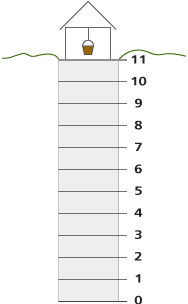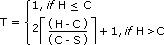# Patterns in Context Homework

## Session 2, Homework

### Problem H1

Look at this table:

 Input Output 1 6 2 10 3 14 4 18 5 22 6 26 ? ? ? ?
1. Find and describe several patterns in the table.
2. If you used a variable in your description, explain its meaning.
3. What is the 100th entry in the table?
4. Is 102 ever an “output”? How do you know?

### Problem H2

Mr. Lewis looked at the table in Problem H1 and said, “Oh, I get it. Just do these steps.”

1. First, you multiply the input number by itself and multiply that answer by 6.
2. Then you add 6 to the answer you just got, and call that number A.
3. Then, start over. Multiply the input number by itself and add 11, and call that B.
4. Multiply the input number by 5, and call that C.
5. Then, multiply B and C together, and divide by 6. Call that D.
6. Finally, subtract D from A, and that’s all!

If you do all this, you’ll get the numbers in the table.” Does Mr. Lewis get it? Does Mr. Lewis’ method agree with yours for the first three outputs? For the next three? Which method is correct?

### Problem H3

A frog climbs up the side of a well and slides back while resting. Every minute the frog leaps forward 5 meters (and it leaps forward precisely at the end of the minute). Then it rests for a minute. At the end of the rest, the frog slips back 3 meters. At the end of the next minute it leaps (5 meters), then a minute later it slides back (3 meters), and so on.

Here is a picture of a well 11 meters deep.You may find it helpful to work with someone to model what’s happening in the problem.

1.  Fill in the table below. Why is there only one correct way to fill in the table?
 Height of well Time it takes to get out 1 2 3 4 5 6 7 8 9 10 11 12 13 14 15 16 17
2. There are other ways to represent situations besides tables. Try drawing a visual image (a graph or a picture) that conveys information about the frog in the well.
3. How long would it take the frog to get out of a well that is 30 meters deep? One that is 100 meters? One that is 103 meters?Tip: Try to predict using a rule rather than extending a table.
4.  The (tired) frog took 13 minutes to get out of the well. How deep could the well have been?Tip: Is there only one answer to this question?

### Problem H4

1. Suppose our frog could climb 6 meters per minute and slid only 2 meters while resting. How long would it take to get out of a 100-meter well?
2. Suppose our frog could climb n meters per minute, and slid only 2 meters while resting. Describe, in terms of n, how long it would take to get out of a 100-meter well.
3. There are several numbers that contribute to the frog’s predicament. There’s the climbing rate (5 meters per minute in the original problem), the slippage rate (originally 3 meters per minute), and the height of the well. Figure out a rule that allows you to calculate the escape time if you are given the climbing rate, the slippage rate, and the height of the well. (Such a rule is called a function — we’ll look at functions in depth later on in the course.)

Tip: Similar reasoning to some of the Eric problems will be helpful here, but this
is a true challenge.

Vance, James. “Number Operations from an Algebraic Perspective.”

Solomon, Jesse, Carol Martignette-Boswell, et al. “Toward a Cooperative Model of Math Staff Development.”
Reproduced with permission from Mathematics Teaching in the Middle School, © 1997 by the National Council of Teachers of Mathematics. All rights reserved.

Chapter 1
Chapter 2

Usiskin, Zalman. “Conceptions of School Algebra and Uses of Variables.”

Chapter 1
Chapter 2

### Solutions

Problem H1

1. Although there are not many entries in the table, one possible pattern is that each output number is 1 more than the square of each input number.
2. One formula is O = n2 + 1, where n is the input number. Others are possible.
3. If the table’s pattern continues as expected, the formula gives O = 1002 + 1 = 10,001. Other answers are possible with other patterns.
4. If the table’s pattern continues, 102 will never appear. The 10th entry will be 101, and the 11th entry will be 122. (Why can’t 102 appear later, or earlier, in the table?) Of course, the table lends itself to many other possible patterns, and some of these may include the number 102. For example, the list may include only those positive numbers that end in 2, 5, or 0, in which case 102 will be the 31st number in the list.

Problem H2

Mr. Lewis is making things a lot more difficult than they need to be! Here’s the analysis on the number n:

1. n * n = n2, multiplied by 6 is 6n2
2. 6n2 plus 6 is (6n2 + 6) = A
3. The result here is n2 + 11 = B
4. The result here is 5n = C
5. B * C = (n2 + 11) * 5n = 5n3 + 55n, divided by 6 gives (5n2 + 55n) / 6 = D
6. The final answer is 6n2 + 6 – (5n3 + 55n) / 6. This value cannot be simplified, because it does not have any variables with the same exponent.Let’s look at a table for Mr. Lewis’s rule for small values of n and compare it to the table for O = n2 + 1.
 Input(n) Output(n2 + 1) Mr. Lewis’s Rule 1 2 6 + 6 – 10 = 2 2 5 24 + 6 – 25 = 5 3 10 54 + 6 – 50 = 10 4 17 96 + 6 – 90 = 12 5 26 150 + 6 – 150 = 6 10 101 600 + 6 – 1750 = -1144

As you can see from the table, Mr. Lewis’s rule generates the three numbers we were given in the table, but begins to veer away from the table’s values quickly. By n = 10 there is a drastic difference in the values from the two rules. This doesn’t make Mr. Lewis’s rule wrong, but it is definitely not the simplest rule that generates the table’s values.

Problem H3

1. Here is the completed table. There is only one way to complete the table, because we are trying to find the shortest time it will take the frog to escape the well. Therefore, there can only be one shortest time to put into the table.
 Height of well Time it takes to get out 1 1 minute 2 1 minute 3 1 4 1 5 3 6 3 7 5 8 5 9 7 10 7 11 9 12 9 13 11 14 11 15 13 16 13 17 15

Three observations: First, the values are all 1 minute until the well is 6 meters deep, because the frog can climb 5 meters in the 1st minute. Second, the times to climb out are all odd numbers, because it takes 2 minutes before the frog will climb again. Third, the times occur in pairs, because the difference between the frog’s jumping distance and sliding distance is 2 meters. This means that it won’t matter if the frog needs to make 6 meters or 7 meters, because it will make either in 2 jumps.

2. A 30-meter climb will take 27 minutes. A 100-meter climb will take 97 minutes. A 103-meter climb will take 99 minutes. Poor frog.
3. According to the table, the well could have been either 16 or 17 meters deep.

Problem H4

1. This extension will change the way the table is constructed. The first 6 meters will all take 1 minute, the next 4 will take 3 minutes, the next 4 will take 5 minutes, and so forth. This happens because the frog gains 4 meters in each 2-minute cycle of jumping and resting. So it will take 24 2-minute cycles for the frog to get within the 6-meter range for its escape, and it will take a total of 49 minutes for the frog to make it all the way out of a 100-meter well.
2. Now the frog will take only 1 minute to escape if the well is n meters or less, and each extra minute the frog can climb (n � 2) more meters. The full formula here requires the greatest integer function, but a quick calculation would be to subtract 2, divide by (n � 2), round the answer up to the nearest whole number, double it, then subtract 1. The subtraction by 2 is done because the frog doesn’t slip right away, and the dividing by (n � 2) is done because it is the total height gained by the frog in one cycle of climbing and slipping. The doubling is done because one cycle takes 2 minutes, and the subtracting by 1 is done because the frog doesn’t slip in the cycle that he manages to escape.
3. Let’s call the climbing rate C, the slippage rate S, and the height H. It’s pretty difficult to create a formula for T, the time for the frog to escape, but first a few observations:
• If the given height H is less than or equal to C, the answer should be 1 minute.
• Every 2 minutes after the first, if the frog is still stuck, it gains (C – S) meters.

This means that we’ll need to calculate (H – C) / (C – S) to find how many 2-minute cycles the frog will need to go through to get within C meters of the top, then 1 more minute to escape. Additionally, because (H – C) / (C – S) often gives a fraction, we have to include instructions to round that number up to the next integer. (This is usually called the “ceiling” function, and is denoted by the oddly shaped brackets seen below. We used this function when we talked about Eric the Sheep.) Additionally (!), because (H – C) might be negative, we have to make an exception for those times that the frog can just hop on out. So, having done all that, here’s the formula for T, the time that it takes the frog to escape:Test it out on the previous problems if you like.